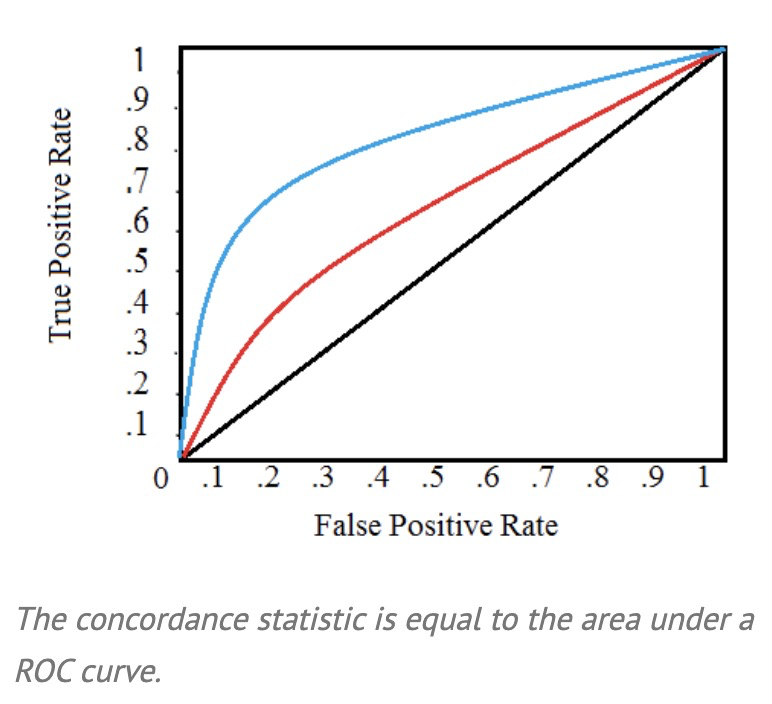# C 统计量 / C statisticGeek_Goldensikaiqi

Abstract:

The C-statistic (sometimes called the “concordance” statistic or C-index) is a measure of goodness of fit for binary outcomes in a logistic regression model.

In clinical studies, the C-statistic gives the probability a randomly selected patient who experienced an event (e.g. a disease or condition) had a higher risk score than a patient who had not experienced the event.

It is equal to the area under the Receiver Operating Characteristic (ROC) curve and ranges from 0.5 to 1.• A value below 0.5 indicates a very poor model.

• A value of 0.5 means that the model is no better than predicting an outcome than random chance.

• Values over 0.7 indicate a good model.

• Values over 0.8 indicate a strong model.

• A value of 1 means that the model perfectly predicts those group members who will experience a certain outcome and those who will not.

The C-statistic isn’t used very often as it only gives you a general idea about a model; A ROC curve contains much more information about accuracysensitivity and specificity.

## Weighting

A weighted c-index is used when the cost of failing to predict a positive outcome (like a test for cancer) is higher than benefit of correctly predicting a negative outcome. Weighting penalizes models that result in small probability differences for positive and negative outcomes, but doesn’t change the value of the C-statistic. It can also be used to adjust for stratified random sampling.

## Statistical Significance

Like most statistics, the C-statistic is sometimes paired with a confidence interval. For example, you might have a result of 0.63 with a confidence interval ranging from 0.53 to 0.73). In general, any result is not significant if it includes 0.5, even if it includes the relevant C-statistic. For example, a result of 0.63 with a CI ranging from 0.43 to 0.83 would not be significant because it includes 0.5 in that range.

Reference:

Stephanie Glen. "C-Statistic: Definition, Examples, Weighting and Significance" From StatisticsHowTo.com: Elementary Statistics for the rest of us! https://www.statisticshowto.com/c-statistic/## 评论